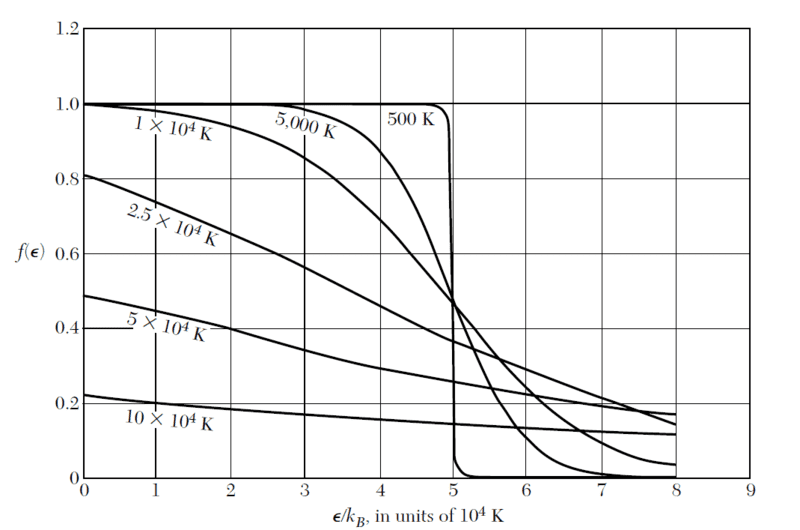# Fermi energy definition and Fermi-Dirac distribution

• I
• chikchok
For T>0 the Fermi-Dirac function gives a smooth decrease from 1 to 0. In summary, Fermi energy is defined as the topmost filled level in the ground state of an N electron system and this definition only holds for absolute zero. For non-absolute zero temperatures, Fermi energy is the energy at which the probability of a state being occupied is 50 percent. The Fermi-Dirac function shows a sharp step from 1 to 0 at low temperatures, indicating that levels below the Fermi level are filled and all above are empty. However, at higher temperatures, the Fermi-Dirac function decreases smoothly. This is due to the fluctuation of occupation levels at finite temperatures, and

#### chikchok

TL;DR Summary
Fermi energy definition and fermi-dirac distribution
1)In my book , there is a definition of fermi energy as topmost filled level in the ground state of an N electron system. This definition holds only for absolute zero,right? If it is not absolute zero,fermi energy is the energy at which the probability of a state being occupied is 50 percent. Please, tell me if I am understanding this correctly.
2)I was wondering why at low temperatures Fermi-Dirac function goes sharply from 1 to 0 and for higher temperature it goes down smoothly. Is it reasonable to assume that for low temperature, levels below the fermi level are filled and all above are empty? But why it does not happen with higher temperatures. Thank you in advance.Last edited by a moderator:
1) Stating "In the ground state" obviates mention of temperature.
2) Temperature is a measure of average internal energy. An isolated system at lowest energy will be in the ground state. If connected to a thermal reservoir it will have a finite probability of being in an excited state with a probability that is roughly negative exponential in energy e.g. the Boltzmann factor. Careful consideration of quantum effects gives rise to Fermi-Dirac statistics for half integer spin which gives a "step" function for T=0

hutchphd said:
1) Stating "In the ground state" obviates mention of temperature.
2) Temperature is a measure of average internal energy. An isolated system at lowest energy will be in the ground state. If connected to a thermal reservoir it will have a finite probability of being in an excited state with a probability that is roughly negative exponential in energy e.g. the Boltzmann factor. Careful consideration of quantum effects gives rise to Fermi-Dirac statistics for half integer spin which gives a "step" function for T=0
so for non-absolute temperature fermi energy is not the energy of topmost filled level anymore?

The Fermi Energy is defined as the zero T result. It is "5" for your graphed system. With finite temperature occupation of levels is fluctuating.

## 1. What is Fermi energy and why is it important in physics?

Fermi energy, also known as Fermi level, is the highest energy state occupied by an electron at absolute zero temperature in a solid material. It represents the energy at which the available energy states are filled by electrons. Fermi energy is important in physics because it determines the electrical and thermal properties of materials, such as conductivity and heat capacity.

## 2. How is Fermi energy related to the Fermi-Dirac distribution?

The Fermi-Dirac distribution is a probability distribution that describes the distribution of electrons in a system at thermal equilibrium. The Fermi energy is the parameter that determines the shape of this distribution, specifically the location of the Fermi level. As the temperature approaches absolute zero, the Fermi-Dirac distribution becomes a step function with a sharp edge at the Fermi energy.

## 3. What is the difference between Fermi energy and Fermi temperature?

Fermi energy and Fermi temperature are two different concepts that are often confused. Fermi energy is a characteristic of a material, while Fermi temperature is a characteristic of a system. Fermi energy is the energy level at which the available energy states are filled, while Fermi temperature is the temperature at which the thermal energy of the system is equal to the Fermi energy.

## 4. How is the Fermi energy affected by changes in temperature and doping?

The Fermi energy is affected by changes in temperature and doping in a material. As the temperature increases, the Fermi energy also increases due to the increased thermal energy of the electrons. Doping, which is the intentional introduction of impurities into a material, can either increase or decrease the Fermi energy depending on the type and concentration of dopants.

## 5. Can the Fermi energy be directly measured in experiments?

The Fermi energy cannot be directly measured in experiments. However, it can be indirectly determined by measuring other properties that are related to the Fermi energy, such as electrical conductivity, specific heat, and thermoelectric power. These measurements can then be used to calculate the Fermi energy through mathematical equations.